Language :

Quant Practice Quiz for SBI Clerk Prelims Exam 2018 - Set 66Question 1 99, 49.5, 50.5, 77.25, 156.5, ? 99, 49.5, 50.5, 77.25, 156.5, ?Read Less
 A 375B 387.5C 302.5D 393.75E None of these
 Question 2 4.5, 5.5, 9.5, 36.5, 52.5, 177.5, ? 4.5, 5.5, 9.5, 36.5, 52.5, 177.5, ?Read Less
 A 205.5B 213.5C 227.5D 236.5E None of these
 Question 3 (20+13) × 25% of (20+12) – 12 × 7% of 225 = ? (20+13) × 25% of (20+12) – 12 × 7% of 225 = ?Read Less
 A 75B 80C 84D 90E None of these
 Question 4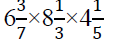Read Less
 A 125B 245C 225D 195E None of these
 Question 5 A person has pure milk at Rs 30 per litre. In what ratio should he mixed some water in the milk, so that after selling the mixture at Rs 25 per litre he may get a profit of 25%? A person has pure milk at Rs 30 per litre. In what ratio should he mixed some water in the milk, so that after selling the mixture at Rs 25 per litre he may get a profit of 25%?Read Less
 A 3 : 2B 12 : 1C 2 : 1D 5 : 2E None of these
 Question 6 Average weight of three boys A,B and C is 40 kg while the average weight of three boys, B, D and E is 50 kg. What is the average weight of A, B, C, D and E Average weight of three boys A,B and C is 40 kg while the average weight of three boys, B, D and E is 50 kg. What is the average weight of A, B, C, D and ERead Less
 A 51 kgB 53 kgC 49 kgD Data inadequateE None of these
 Question 7 What will be the compound interest accrued on an amount. Rs. 10,000 at the rate of 20% p.a. in two years if the interest is compounded half yearly? What will be the compound interest accrued on an amount. Rs. 10,000 at the rate of 20% p.a. in two years if the interest is compounded half yearly?Read Less
 A Rs. 4,200B Rs. 4,440C Rs. 4,550D Rs. 4,641E None of these
 Question 8 While selling a mobile, a shopkeeper gives a discount of 15%. If he gives a discount of 20%, he earns Rs. 500 less as profit. What is the        marked price of the mobile While selling a mobile, a shopkeeper gives a discount of 15%. If he gives a discount of 20%, he earns Rs. 500 less as profit. What is the        marked price of the mobileRead Less
 A Rs. 9200B Rs. 9800C Rs. 10000D Rs. 12500E None of these
 Question 9 On an amount of Rs. 10,000, a person has choice between 3 successive discounts of 25%, 10% and 30%, and 3 successive discounts of 20%, 20% and 15%. Choose the better one and find how much he can save : On an amount of Rs. 10,000, a person has choice between 3 successive discounts of 25%, 10% and 30%, and 3 successive discounts of 20%, 20% and 15%. Choose the better one and find how much he can save :Read Less
 A Rs. 715B Rs. 710C Rs. 130D Rs. 690E None of these
 Question 10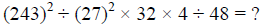Read Less
 A 192B 216C 230D 244E 256
 Question 11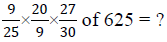Read Less
 A 350B 375C 425D 450E None of these
 Question 12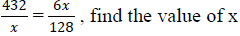Read Less
 A 84B 96C 108D 112E None of these
 Question 13 58.33% of 2448/6 + 62.5% of 360 = 18 × ? 58.33% of 2448/6 + 62.5% of 360 = 18 × ?Read Less
 A 15B 20C 26D 32E None of these
 Question 14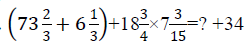Read Less
 A 181B 164C 175D 210E None of these
 A 16B 14C 15D 10E None of these

## SBI Clerk - ONLINE TEST

IBT Institute offer you the most trusted and highly recommended Test Series for SBI Clerk Exam. 8 out of every 10 candidates selected in SBI Clerk last year opted for IBT Online Test Series.

## SBI Clerk - CLASS ROOM COURSE

Keeping in mind the SBI Clerk Exam, IBT has launched Special batches for SBI Clerk Exam to guide you through your preparations. Seats filling fast. Don’t miss out!!Correspondence Course

For SBI PO Exam (Pre+Main)

9 Books , 350 Tests

52 Assignments , Online Doubts